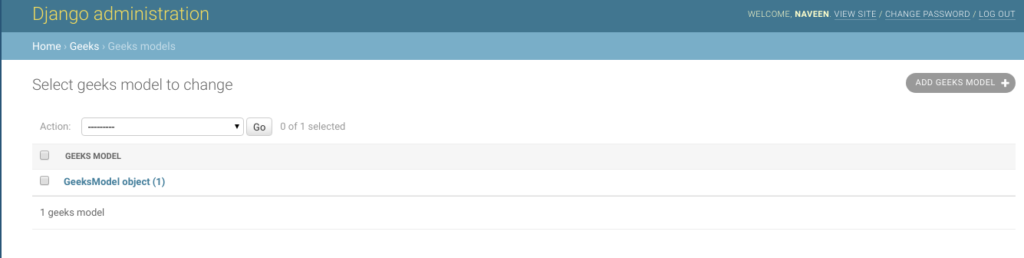# DecimalField – Django Models

• Last Updated : 12 Feb, 2020

DecimalField is a field which stores a fixed-precision decimal number, represented in Python by a Decimal instance. It validates the input using DecimalValidator.

Syntax

Attention geek! Strengthen your foundations with the Python Programming Foundation Course and learn the basics.

To begin with, your interview preparations Enhance your Data Structures concepts with the Python DS Course. And to begin with your Machine Learning Journey, join the Machine Learning - Basic Level Course

`field_name = models.DecimalField(max_digits=None, decimal_places=None, **options)`
• #### DecimalField.max_digits

The maximum number of digits allowed in the number. Note that this number must be greater than or equal to decimal_places.

• #### DecimalField.decimal_places

The number of decimal places to store with the number.

For example, to store numbers up to 999 with a resolution of 2 decimal places, you’d use:

`models.DecimalField(..., max_digits=5, decimal_places=2)`

And to store numbers up to approximately one billion with a resolution of 10 decimal places:

`models.DecimalField(..., max_digits=19, decimal_places=10)`

## Django Model DecimalField Explanation

Illustration of DecimalField using an Example. Consider a project named `geeksforgeeks` having an app named `geeks`.

Refer to the following articles to check how to create a project and an app in Django.

Enter the following code into `models.py` file of geeks app.

 `from` `django.db ``import` `models``from` `django.db.models ``import` `Model``# Create your models here.`` ` `class` `GeeksModel(Model):``    ``geeks_field ``=` `models.DecimalField(``                         ``max_digits ``=` `5``,``                         ``decimal_places ``=` `2``)`

Add the geeks app to `INSTALLED_APPS`

 `# Application definition`` ` `INSTALLED_APPS ``=` `[``    ``'django.contrib.admin'``,``    ``'django.contrib.auth'``,``    ``'django.contrib.contenttypes'``,``    ``'django.contrib.sessions'``,``    ``'django.contrib.messages'``,``    ``'django.contrib.staticfiles'``,``    ``'geeks'``,``]`

Now when we run `makemigrations` command from the terminal,

`Python manage.py makemigrations`

A new folder named migrations would be created in `geeks` directory with a file named `0001_initial.py`

 `# Generated by Django 2.2.5 on 2019-09-25 06:00`` ` `from` `django.db ``import` `migrations, models`` ` `class` `Migration(migrations.Migration):`` ` `    ``initial ``=` `True`` ` `    ``dependencies ``=` `[``    ``]`` ` `    ``operations ``=` `[``        ``migrations.CreateModel(``            ``name ``=``'GeeksModel'``,``            ``fields ``=``[``                ``(``'id'``, ``                  ``models.AutoField(``                  ``auto_created ``=` `True``,``                  ``primary_key ``=` `True``,``                  ``serialize ``=` `False``, ``                  ``verbose_name ``=``'ID'``                ``)),``                ``(``'geeks_field'``, models.DecimalField(``                                        ``max_digits ``=` `5``,``                                        ``decimal_places ``=` `2``                ``)),``            ``],``        ``),``    ``]`

Now run,

`Python manage.py migrate`

Thus, an` geeks_field `DecimalField is created when you run migrations on the project. It is a field to store `datetime.date` python object.

## How to use DecimalField ?

DecimalField is used for storing python datetime.date instance in the database. One can store any type of decimal number in the database. Let’s try storing a decimal number in the model created above.

 `# importing the model``# from geeks app``from` `geeks.models ``import` `GeeksModel`` ` `# importing datetime module``import` `decimal`` ` `# creating an instance of ``# datetime.date``d ``=` `decimal.Decimal(``9.53``)`` ` `# creating an instance of ``# GeeksModel``geek_object ``=` `GeeksModel.objects.create(geeks_field ``=` `d)``geek_object.save()`

Now let’s check it in admin server. We have created an instance of GeeksModel.## Field Options

Field Options are the arguments given to each field for applying some constraint or imparting a particular characteristic to a particular Field. For example, adding an argument `null = True` to DecimalField will enable it to store empty values for that table in relational database.
Here are the field options and attributes that a DecimalField can use.

Field OptionsDescription
NullIf True, Django will store empty values as NULL in the database. Default is False.
BlankIf True, the field is allowed to be blank. Default is False.
db_columnThe name of the database column to use for this field. If this isn’t given, Django will use the field’s name.
DefaultThe default value for the field. This can be a value or a callable object. If callable it will be called every time a new object is created.
help_textExtra “help” text to be displayed with the form widget. It’s useful for documentation even if your field isn’t used on a form.
primary_keyIf True, this field is the primary key for the model.
editableIf False, the field will not be displayed in the admin or any other ModelForm. They are also skipped during model validation. Default is True.
error_messagesThe error_messages argument lets you override the default messages that the field will raise. Pass in a dictionary with keys matching the error messages you want to override.
help_textExtra “help” text to be displayed with the form widget. It’s useful for documentation even if your field isn’t used on a form.
verbose_nameA human-readable name for the field. If the verbose name isn’t given, Django will automatically create it using the field’s attribute name, converting underscores to spaces.
validatorsA list of validators to run for this field. See the validators documentation for more information.
UniqueIf True, this field must be unique throughout the table.

My Personal Notes arrow_drop_up